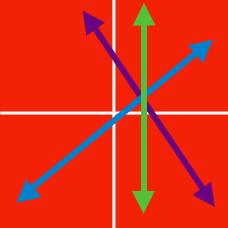Algebra

# System of Linear Equations: Level 1 Challenges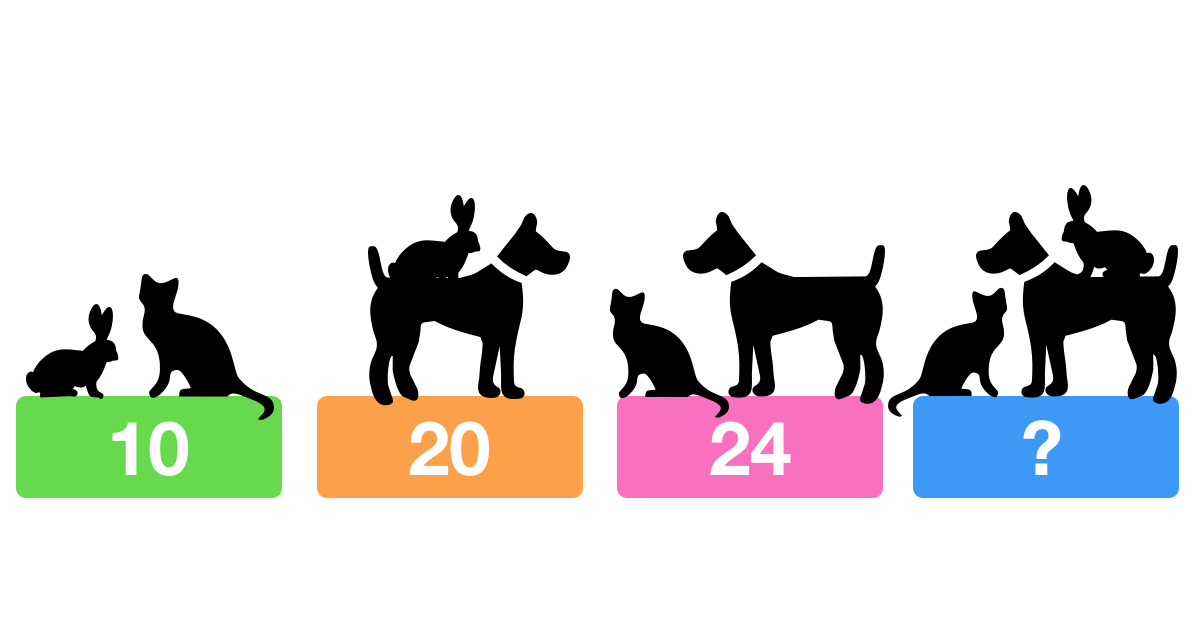What weight will the fourth scale display?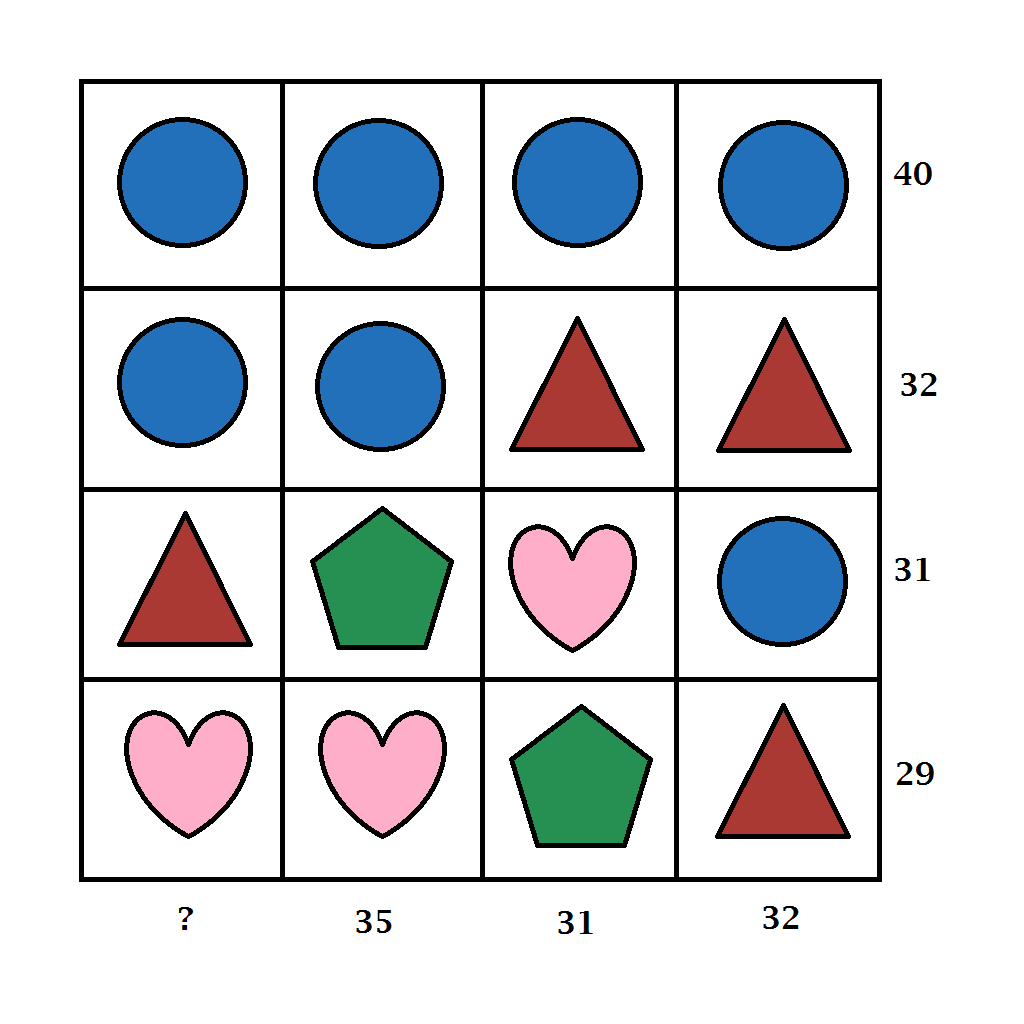If the numbers alongside each column and row are the total of the values of the symbols within that column and row, then which number should replace the question mark?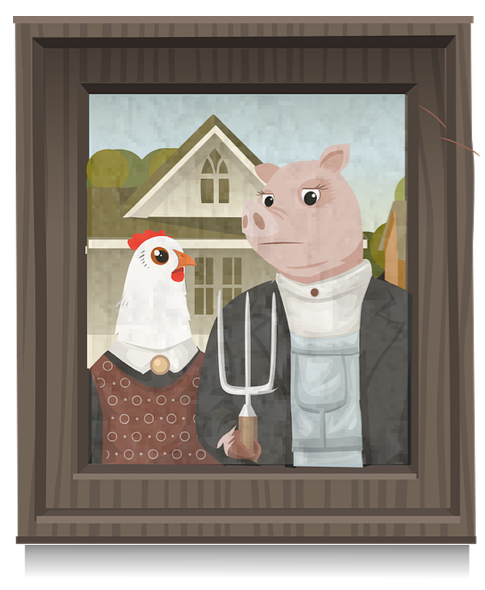A pen is filled with chickens and pigs.

There are 240 feet and 82 heads in the pen.

How many pigs are there?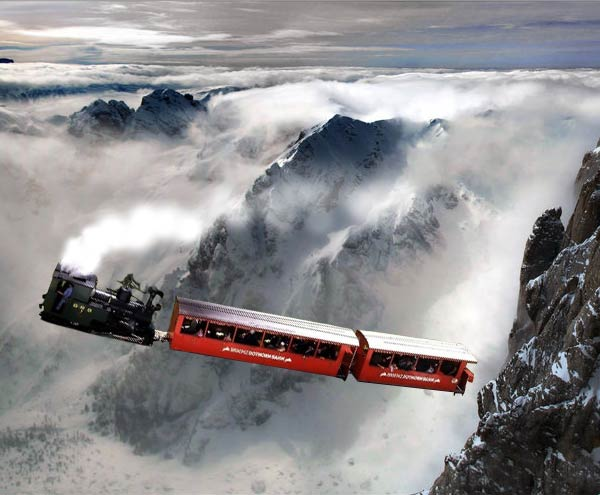A train covers a certain distance at a uniform speed. If the train had been 6 km/hr faster, it would have taken four hours less than the scheduled time. And if the train were slower by 6 km/hr, the train would have taken six hours more than the scheduled time. Find the length of the journey in kilometers.I have some pencils and some jars.
If I put 4 pencils into each jar, I will have one jar left over.
If I put 3 pencils into each jar, I will have one pencil left over.
How many pencils and how many jars do I have?

×